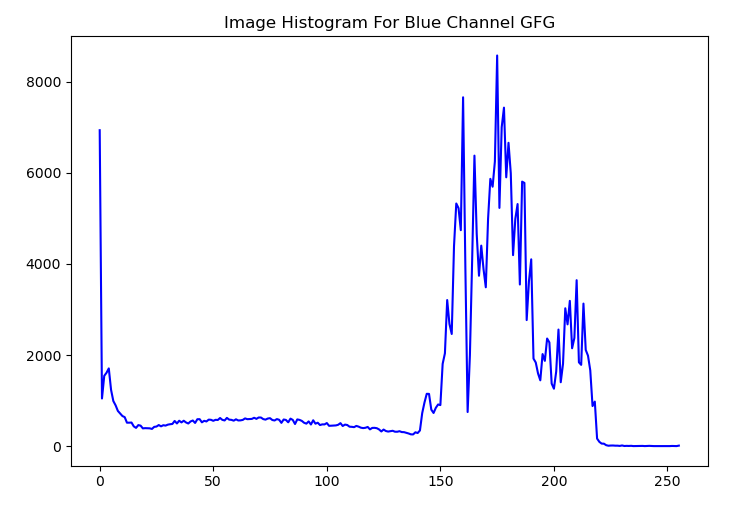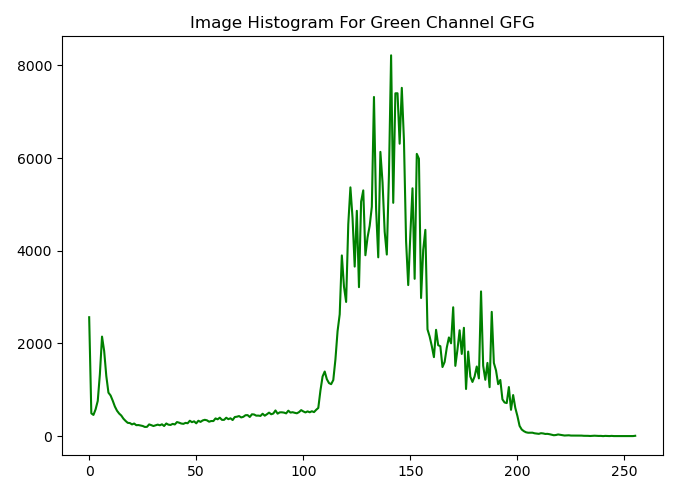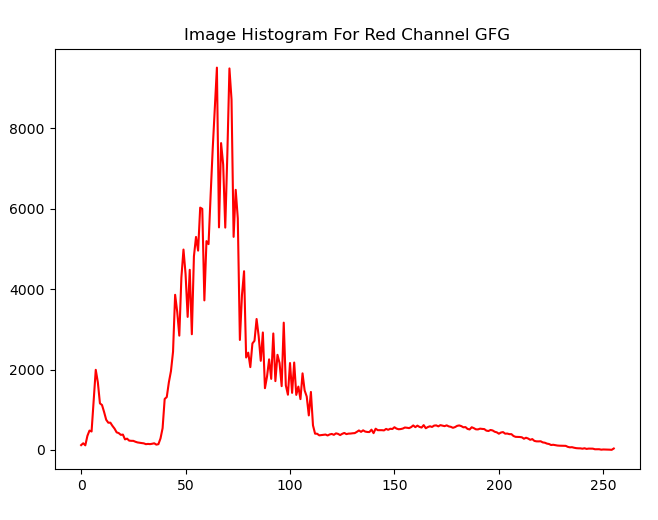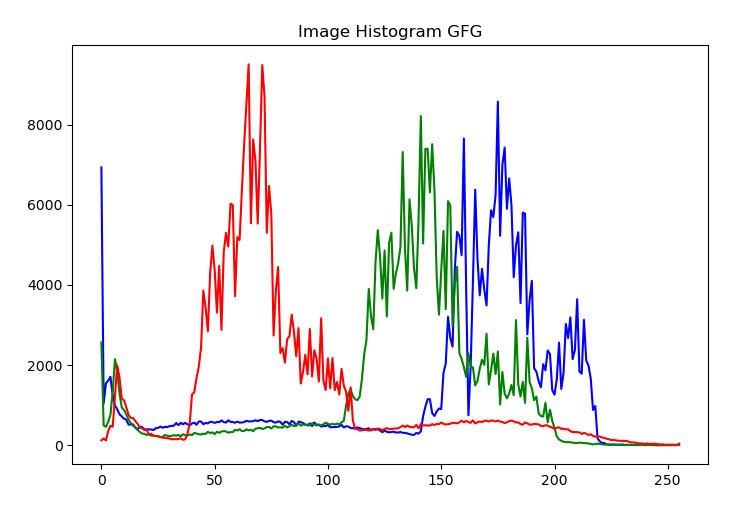# Python OpenCV – cv2.calcHist method

• Last Updated : 28 Feb, 2022

OpenCV provides us with the cv2.calcHist() function to calculate the image histograms. We could apply it to calculate the histogram of the constituent color channels (blue, green, and red) of the image. When we read the image using cv2.imread() method, the image read is in BGR format. We could use the below syntax for the cv2.calcHist() function.

cv2.calcHist() function:

Syntax: cv2.calcHist(images, channels, mask, histSize, ranges[, hist[, accumulate]])

Parameters:

• images: list of images as numpy arrays. All images must be of the same dtype and same size.
• channels: list of the channels used to calculate the histograms.
• mask: optional mask (8 bit array) of the same size as the input image.
• histSize: histogram sizes in each dimension
• ranges: Array of the dims arrays of the histogram bin boundaries in each dimension
• hist: Output histogram
• accumulate: accumulation flag, enables to compute a single histogram from several sets of arrays.

Return: It returns an array of histogram points of dtype float32.

Let’s understand the cv2.calcHist() function with the help of some Python examples.

The following image is used as an input image in the examples below.### Example 1:

In this example, we calculate the histogram of the blue color channel of the input image “mountain.jpg” using cv2.calcHist() function.  We pass the parameter channels =  to calculate the histogram of the blue channel. We also plot the histogram using Matplotlib.

## Python3

 `# Python program to compute and visualize the``# histogram of Blue channel of image``%``matplotlib inline`` ` `# importing libraries``import` `cv2``import` `numpy as np``from` `matplotlib ``import` `pyplot as plt`` ` `# reading the input image``img ``=` `cv2.imread(``'mountain.jpg'``)`` ` `# computing the histogram of the blue channel of the image``hist ``=` `cv2.calcHist([img],[``0``],``None``,[``256``],[``0``,``256``])`` ` `# plot the above computed histogram``plt.plot(hist, color``=``'b'``)``plt.title(``'Image Histogram For Blue Channel GFG'``)``plt.show()`

Output:### Example 2:

In this example, we calculate the histogram of the green color channel of the input image “mountain.jpg” using cv2.calcHist() function.  We pass the parameter channels =  to calculate the histogram of the green channel. We also plot the histogram using Matplotlib.

## Python3

 `# Python program to compute and visualize the``# histogram of Green channel of image``%``matplotlib inline`` ` `# importing libraries``import` `cv2``import` `numpy as np``from` `matplotlib ``import` `pyplot as plt`` ` `# reading the input image``img ``=` `cv2.imread(``'mountain.jpg'``)`` ` `# computing the histogram of the green channel of the image``hist ``=` `cv2.calcHist([img],[``1``],``None``,[``256``],[``0``,``256``])`` ` `# plot the above computed histogram``plt.plot(hist, color``=``'g'``)``plt.title(``'Image Histogram For Green Channel GFG'``)``plt.show()`

Output:### Example 3:

In this example, we calculate the histogram of the red color channel of the input image “mountain.jpg” using cv2.calcHist() function.  We pass the parameter channels =  to calculate the histogram of the red channel. We also plot the histogram using Matplotlib.

## Python3

 `# Python program to compute and visualize the``# histogram of Red channel of image``%``matplotlib inline`` ` `# importing libraries``import` `cv2``import` `numpy as np``from` `matplotlib ``import` `pyplot as plt`` ` `# reading the input image``img ``=` `cv2.imread(``'mountain.jpg'``)`` ` `# computing the histogram of the Red channel of the image``hist ``=` `cv2.calcHist([img],[``2``],``None``,[``256``],[``0``,``256``])`` ` `# plot the above computed histogram``plt.plot(hist, color``=``'r'``)``plt.title(``'Image Histogram For Red Channel GFG'``)``plt.show()`

Output:### Example 4:

In this example, we calculate the histogram of all three color channels of the input image “mountain.jpg” using cv2.calcHist() function.  We use a for loop to iterate over all three color channels. We also plot the histogram using Matplotlib.

## Python3

 `# Python program to compute and visualize the``# histogram of image for all three channels``%``matplotlib inline`` ` `# importing libraries``import` `cv2``import` `numpy as np``from` `matplotlib ``import` `pyplot as plt`` ` `# reading the input image``img ``=` `cv2.imread(``'mountain.jpg'``)`` ` `# define colors to plot the histograms``colors ``=` `(``'b'``,``'g'``,``'r'``)`` ` `# compute and plot the image histograms``for` `i,color ``in` `enumerate``(colors):``    ``hist ``=` `cv2.calcHist([img],[i],``None``,[``256``],[``0``,``256``])``    ``plt.plot(hist,color ``=` `color)``plt.title(``'Image Histogram GFG'``)``plt.show()`

Output:My Personal Notes arrow_drop_up